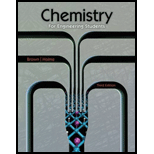# According to the U.S. Nuclear Regulatory Commission, in 2011 there were 104 commercial nuclear reactors in the United States. In 2011, 4.1 10 6 gigawatt-hours of electricity were generated for use, and 19.43% of that was generated by nuclear power. (a) A nuclear technician assumed that the products of the fission of 2 3 5 U by one neutron were 9 7 Zr and 1 3 7 Te (see Problem 14.55). Ignoring decay products, the technician estimated the total mass of zirconium and tellurium in metric tons that would have to be disposed of in 2011 as nuclear waste (l watt-hour = joules; 1 metric ton = kg). What was the technician's result? (b) Look up the half-lives of 9 7 Zr and 1 3 7 Te. When the waste was disposed of, do you think there was a measurable quantity of either isotope? Explain. (c) Look up the decay mode of each isotope and write the decay series to a stable isotope for each one. Which decay series has a radioactive isotope that will remain in the waste for many years? (d) Using the stable isotope products in your calculation, how far off was the technician k estimate of total waste? Explain.### Chemistry for Engineering Students

3rd Edition
Lawrence S. Brown + 1 other
Publisher: Cengage Learning
ISBN: 9781285199023### Chemistry for Engineering Students

3rd Edition
Lawrence S. Brown + 1 other
Publisher: Cengage Learning
ISBN: 9781285199023
Chapter 14, Problem 14.59PAE
Textbook Problem
1 views

## According to the U.S. Nuclear Regulatory Commission, in 2011 there were 104 commercial nuclear reactors in the United States. In 2011, 4.1106 gigawatt-hours of electricity were generated for use, and 19.43% of that was generated by nuclear power.(a) A nuclear technician assumed that the products of the fission of 235U by one neutron were 97Zr and 137Te (see Problem 14.55). Ignoring decay products, the technician estimated the total mass of zirconium and tellurium in metric tons that would have to be disposed of in 2011 as nuclear waste (l watt-hour = joules; 1 metric ton = kg). What was the technician's result?(b) Look up the half-lives of 97Zr and 137Te. When the waste was disposed of, do you think there was a measurable quantity of either isotope? Explain.(c) Look up the decay mode of each isotope and write the decay series to a stable isotope for each one. Which decay series has a radioactive isotope that will remain in the waste for many years?(d) Using the stable isotope products in your calculation, how far off was the technician k estimate of total waste? Explain.

Interpretation Introduction

a) Interpretation:

The total estimated mass Zr and Te disposed as nuclear waste by the nuclear technician

Concept Introduction: ${}^{235}U$

(uranium) undergoes a fission reaction which is neutron induced. On doing so, it will split into two smaller nuclei ${}^{97}Zr$

(zirconium) and ${}^{137}Te$(tellurium). In the process, neutrons and gamma rays are released. The nuclear equation for this reaction is as follows:

${}_{92}^{235}U{+}_{0}^{1}n{\to }_{52}^{137}Te{+}_{40}^{97}Zr+{2}_{0}^{1}n$

### Explanation of Solution

The total amount of electrical energy generated by all the nuclear reactors is

${E}_{n}=\frac{19.43}{100}×4.1×{10}^{6}×{10}^{9}×3600=2.867868×{10}^{18}J$

From the problem 14.55, we know the amount of energy released during the fission of 1 kg of ${}^{235}U$ is $8.789748369×{10}^{14}J$

Hence,

Total mass of ${}^{235}U$ used =$\frac{2.867868×{10}^{18}}{8...}$

Interpretation Introduction

(b) Interpretation:

Whether any of the products Zr and Te obtained from the fission of ${}^{235}U$

are available in measurable quantities in the nuclear waste

Concept Introduction: ${}^{235}U$ (uranium) undergoes a fission reaction which is neutron induced. On doing so, it will split into two smaller nuclei ${}^{97}Zr$ (zirconium) and ${}^{137}Te$ (tellurium). In the process, neutrons and gamma rays are released. The nuclear equation for this reaction is as follows:

${}_{92}^{235}U{+}_{0}^{1}n{\to }_{52}^{137}Te{+}_{40}^{97}Zr+{2}_{0}^{1}n$

Interpretation Introduction

(c) Interpretation:

Decay series of each isotope ${}^{97}Zr$ and ${}^{137}Te$

Concept Introduction: ${}^{235}U$ (uranium) undergoes a fission reaction which is neutron induced. On doing so, it will split into two smaller nuclei ${}^{97}Zr$(zirconium) and ${}^{137}Te$ (tellurium). In the process, neutrons and gamma rays are released. The nuclear equation for this reaction is as follows:

${}_{92}^{235}U{+}_{0}^{1}n{\to }_{52}^{137}Te{+}_{40}^{97}Zr+{2}_{0}^{1}n$

Interpretation Introduction

(d) Interpretation:

The difference between the total mass of the waste using the stable isotope products and the technician’s estimated total mass.

Concept Introduction: ${}^{235}U$ (uranium) undergoes a fission reaction which is neutron induced. On doing so, it will split into two smaller nuclei ${}^{97}Zr$ (zirconium) and ${}^{137}Te$ (tellurium). In the process, neutrons and gamma rays are released. The nuclear equation for this reaction is as follows:

${}_{92}^{235}U{+}_{0}^{1}n{\to }_{52}^{137}Te{+}_{40}^{97}Zr+{2}_{0}^{1}n$

### Still sussing out bartleby?

Check out a sample textbook solution.

See a sample solution

#### The Solution to Your Study Problems

Bartleby provides explanations to thousands of textbook problems written by our experts, many with advanced degrees!

Get Started

Find more solutions based on key concepts
Name the following compounds. a. b. c.

Chemistry: An Atoms First Approach

What is the structure and function of the cytoskeleton?

Biology: The Dynamic Science (MindTap Course List)

Most children who die of malnutrition starve to death.

Nutrition: Concepts and Controversies - Standalone book (MindTap Course List)

Match the term listed in Column A with its definition from Column B

Nutrition Through the Life Cycle (MindTap Course List)

For the pyramid in Problem 13, what is the electric flux through one of the four walls?

Physics for Scientists and Engineers: Foundations and Connections

How do you think oxygen reaches the deep ocean for use by the animals living there?

Oceanography: An Invitation To Marine Science, Loose-leaf Versin

(a) Suppose you select four cards at random from a standard deck of playing cards and end up with a macrostate ...

Physics for Scientists and Engineers, Technology Update (No access codes included)